# Equilateral triangle v3

Calculate the content of the colored gray part. Equilateral triangle has side length 8 cm. Arc centers are the vertices of a triangle.

Correct result:

S =  2.5801 cm2

#### Solution: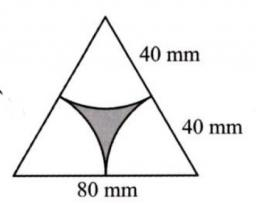We would be pleased if you find an error in the word problem, spelling mistakes, or inaccuracies and send it to us. Thank you!Tips to related online calculators

#### You need to know the following knowledge to solve this word math problem:

We encourage you to watch this tutorial video on this math problem:

## Next similar math problems:

• Soccer balls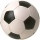Pupils in one class want to buy two soccer balls together. If each of them brings 12.50 euros, they will miss 100 euros, if each brings 16 euros, they will remain 12 euros. How many students are in the class?
• The string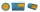They cut 113 cm from the string and divided the rest in a ratio of 5: 6.5: 8: 9.5. The longest part measured 38 cm. Find the original length of the string.
• Pascal's law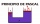Please calculate according to Pascal's law. Krupp's machines were known for their large size. In 1861, a blacksmith's steam hydraulic press was put into operation in Essen. What was the cross-sectional area of the larger piston if a compressive force of 1
• GardensThe area of the square garden is 3/4 of the area of the triangular garden with sides of 80 m, 50 m, 50 m. How many meters of the fence do we need to fence a square garden?
• The staircaseThe staircase has a total height of 3.6 m and forms an angle of 26° with the horizontal. Calculate the length of the whole staircase.
• Railway embankmentThe section of the railway embankment is an isosceles trapezoid, the sizes of the bases of which are in the ratio 5: 3. The arms have a length of 5 m and the height of the embankment is 4.8 m. Calculates the size of the embankment section area.
• A cliff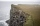A line from the top of a cliff to the ground passes just over the top of a pole 5 ft high and meets the ground at a point 8 ft from the base of the pole. If the point is 93 ft from the base of the​ cliff, how high is the​ cliff?
• Isosceles triangleCalculate the area of an isosceles triangle, the base of which measures 16 cm and the arms 10 cm.
• Right triangleA right triangle ABC is given, c is a hypotenuse. Find the length of the sides a, b, the angle beta if c = 5 and angle alfa = A = 35 degrees.
• Find the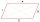Find the content of a regular 12 sided polygon, if its side a = 12 cm.
• Vertical rodThe vertical one meter long rod casts a shadow 150 cm long. Calculate the height of a column whose shadow is 36 m long at the same time.The nine-meter poplar casts a shadow 16.2 m long. How long does a shadow cast by Peter at the same time, if it is 1.4 m high?A sphere is inscribed in the cone (the intersection of their boundaries consists of a circle and one point). The ratio of the surface of the ball and the contents of the base is 4: 3. A plane passing through the axis of a cone cuts the cone in an isosceleCalculate the height of the factory chimney, which casts a shadow 6.5 m long in the afternoon. At the same time, a 6 m high tree standing near it casts a shadow 25 dm long.Triangle A'B'C 'is similar to triangle ABC, whose sides are 5 cm, 8 cm, and 7 cm long. What is the length of the sides of the triangle A'B'C ' if its circumference is 80 cm?In the triangle DEF is DE = 21cm, EF = 14.7cm, DF = 28cm. The triangle D´E´F´ is similar to the triangle DEF. Calculate the lengths of the sides of the triangle D´E´F´ if the similarity coefficient is one-seventh.In the triangle TMA the length of the sides is t = 5cm, m = 3.5cm, a = 6.2cm. Another similar triangle has side lengths of 6.65 cm, 11.78 cm, 9.5 cm. Determine the similarity coefficient of these triangles and assign similar sides to each other.